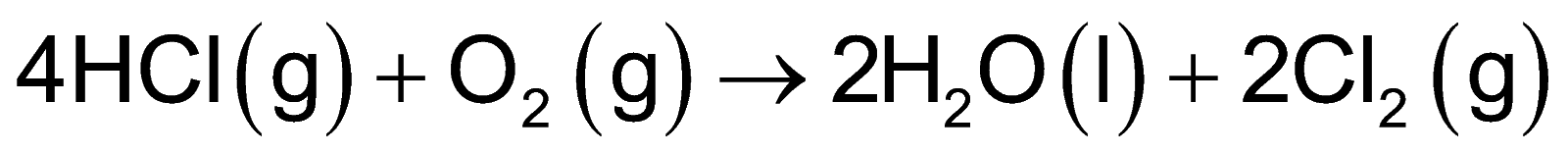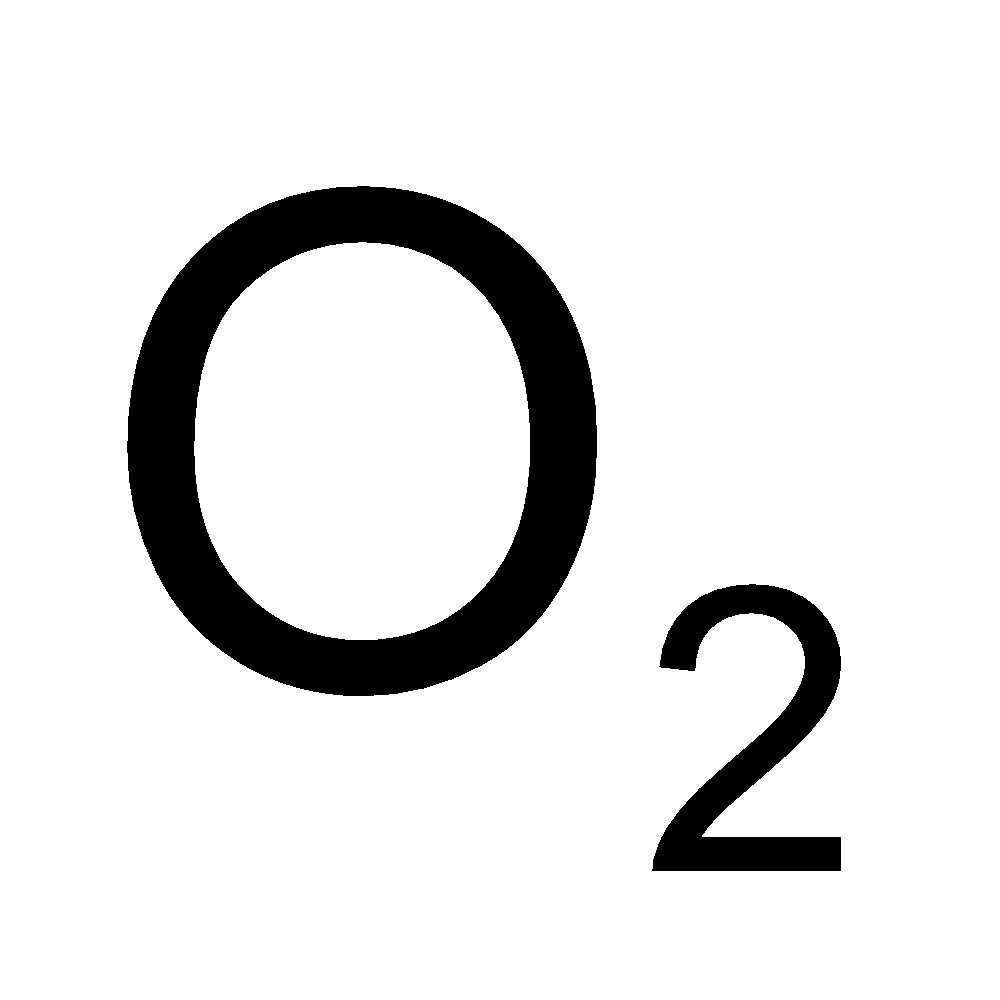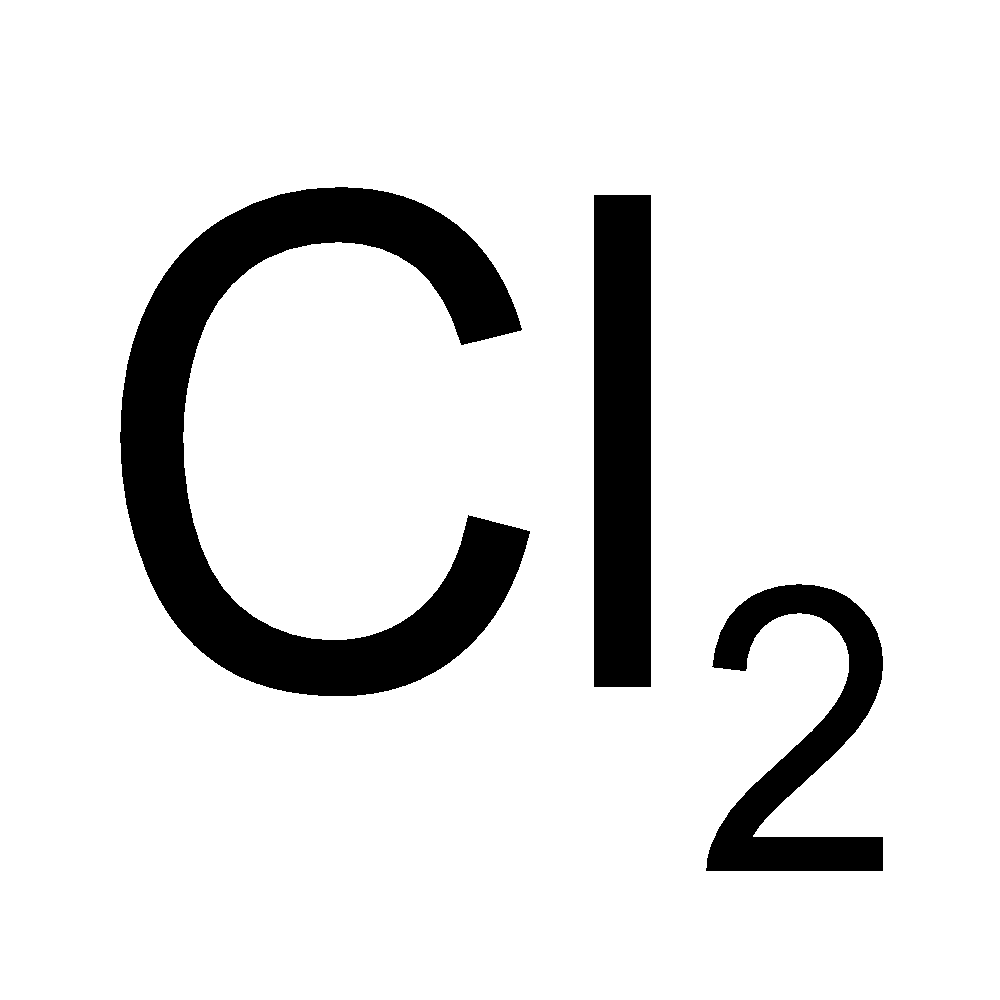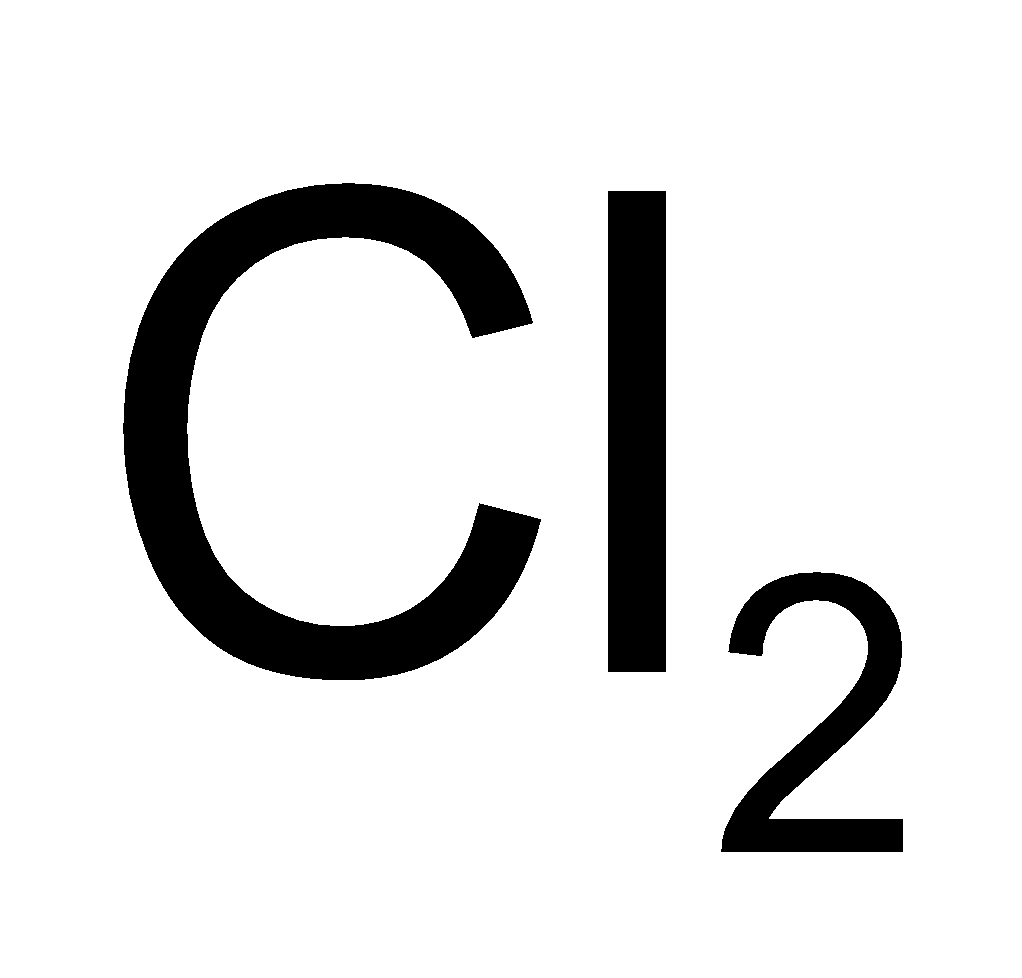×
Get Full Access to Introductory Chemistry - 5 Edition - Chapter 8 - Problem 64p
Get Full Access to Introductory Chemistry - 5 Edition - Chapter 8 - Problem 64p

×

# Consider the reaction between HCl and O2:4 HCl(g) + O2(g) ? 2 H2O(l) + 2 Cl2(g)When 63.1ISBN: 9780321910295 34

## Solution for problem 64P Chapter 8

Introductory Chemistry | 5th Edition

• Textbook Solutions
• 2901 Step-by-step solutions solved by professors and subject experts
• Get 24/7 help from StudySoup virtual teaching assistantsIntroductory Chemistry | 5th Edition

4 5 1 300 Reviews
20
3
Problem 64P

PROBLEM 64P

Consider the reaction between HCl and O2:

4 HCl(g) + O2(g) → 2 H2O(l) + 2 Cl2(g)

When 63.1 g of HCl react with 17.2 g of O2, 49.3 g of Cl2 are collected. Determine the limiting reactant, theoretical yield of Cl2, and percent yield for the reaction.

Step-by-Step Solution:

Problem 64P

Consider the reaction between HCl and O2:When 63.1 g of HCl react with 17.2 g of, 49.3 g ofare collected. Determine the limiting reactant, theoretical yield of, and percent yield for the reaction.

Step by step solution

Step 1 of 7

In any reaction, the reactant species having smaller quantity basically decides the reaction’s yield and such reactant species is designated as “limiting reactant”. In any reaction, how much product gets produced can be calculated using the limiting reactant concept.

The given mass of HCl is 63.1 g.

The given mass ofis 17.2 g.

The actual yield ofis 49.3 g.

Step 2 of 7

Step 3 of 7

##### ISBN: 9780321910295

The full step-by-step solution to problem: 64P from chapter: 8 was answered by , our top Chemistry solution expert on 05/06/17, 06:45PM. Introductory Chemistry was written by and is associated to the ISBN: 9780321910295. The answer to “Consider the reaction between HCl and O2:4 HCl(g) + O2(g) ? 2 H2O(l) + 2 Cl2(g)When 63.1 g of HCl react with 17.2 g of O2, 49.3 g of Cl2 are collected. Determine the limiting reactant, theoretical yield of Cl2, and percent yield for the reaction.” is broken down into a number of easy to follow steps, and 46 words. This textbook survival guide was created for the textbook: Introductory Chemistry, edition: 5. This full solution covers the following key subjects: HCL, reaction, Yield, react, limiting. This expansive textbook survival guide covers 19 chapters, and 2046 solutions. Since the solution to 64P from 8 chapter was answered, more than 520 students have viewed the full step-by-step answer.

Unlock Textbook Solution